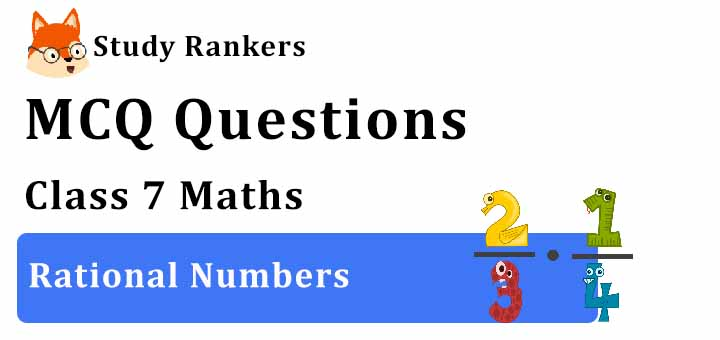>

## MCQ Questions for Class 7 Maths: Ch 9 Rational Numbers1. Rewrite −44/72 in the simplest form.
(a) -18/11
(b) -11/18
(c) -11/19
(d) -11/20
► (c) -11/19

2. The reciprocal of – 5 is ________
(a) 5
(b) -5
(c) -1/5
(d) None of these
► (c) -1/5

3. The product of two numbers is -20/9. If one of the numbers is 4, find the other.
(a) –5/9
(b) 3/11
(c) 12/39
(d) –9/11
► (a) –5/9

4. Write the rational number whose numerator is 4 × (– 7) and denominator is (3 –7) × (15 – 11).
(a) 16/28
(b) 8/13
(c) 13/8
(d) 28/16
► (d) 28/16

5. Which of the following pairs represent the same rational number?
(a) −7/21 and 1/3
(b) 1/3 and −1/9
(c) −5/−9 and 5/−9
(d) 8/−5 and −24/15
► (d) 8/−5 and −24/15

6. Which of the following is not a rational number(s)?
(a) −2/9
(b) 4/−7
(c) −3/−17
(d) √2/3
► (d) √2/3

7. The numbers ________ and ________ are their own reciprocals.
(a) -1 and 0
(b) 1 and 0
(c) 1 and -1
(d) None of these
► (c) 1 and -1

8. Which is the correct descending order of −2,4/−5,−11/20,3/4?
(a) 3/4 > −2 > −11/20 > 4/−5
(b) 3/4 > −11/20 > −4/5 > −2
(c) 3/4 > 4/−5 > −2 > −11/20
(d) 3/4 > 4/−5 >−11/20 > −2
► (b) 3/4 > −11/20 > −4/5 > −2

9. The product of two rational numbers is always a _____.
(a) integer
(b) rational number
(c) natural number
(d) whole number
► (b) rational number

10. Associative property is not followed in _____
(a) integers
(b) whole numbers
(c) rational numbers
(d) natural numbers
► (d) natural numbers

11. Which of the rational number is positive?
(a) 3/7
(b) -5/7
(c) -4/7
(d) -3/7
► (a) 3/7

12. What is the result of 2 − 11/39 + 5/26?
(a) 149/39
(b) 149/78
(c) 149/76
(d) 149/98
► (b) 149/78

13. ____ is the identity for the addition of rational numbers.
(a) 0
(b) 1
(c) -1
(d) None of these
► (a) 0

14. The rational number 9/1 in integer is _____.
(a) 0
(b) 9
(c) -9
(d) 1
► (b) 9

15. Reduce  − 63/99  to the standard form.
(a) 11/7
(b) -7/11
(c) ​7/11
(d) None of these
► (b) -7/11

16. If −4/7 = −32/x, what is the value of x?
(a) 56
(b) −56
(c) ​46
(d) −46
► (a) 56

17. What number should be added to (7/12) to get (4/15) ?
(a) -19/60
(b) -19
(c) 1/2
(d) None of these
► (a) -19/60

18. What is the average of the two middle rational numbers if 4/7,1/3,2/5 and 5/9 are arranged in ascending order?
(a) 80/90
(b) 86/45
(c) 43/45
(d) 43/90
► (d) 43/90